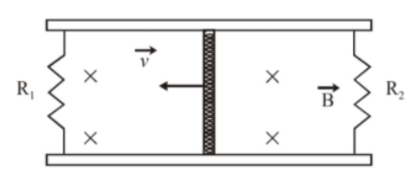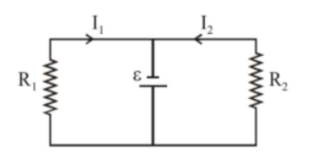# A conducting bar of length $L$ is free to slide on two parallel conducting rails as shown in the figureQuestion:

A conducting bar of length $L$ is free to slide on two parallel conducting rails as shown in the figureTwo resistors $\mathrm{R}_{1}$ and $\mathrm{R}_{2}$ are connected across the ends of the rails. There is\

a uniform magnetic field $\overrightarrow{\mathrm{B}}$ pointing into the page. An external agent pulls the bar to the left at a constant speed $v$

The correct statement about the directions of induced currents $\mathrm{I}_{1}$ and $\mathrm{I}_{2}$

flowing through $\mathrm{R}_{1}$ and $\mathrm{R}_{2}$ respectively is:

1. (1) Both $\mathrm{I}_{1}$ and $\mathrm{I}_{2}$ are in anticlockwise direction

2. (2) Both $\mathrm{I}_{1}$ and $\mathrm{I}_{2}$ are in clockwise direction

3. (3) $\mathrm{I}_{1}$ is in clockwise direction and $\mathrm{I}_{2}$ is in anticlockwise direction

4. (4) $\mathrm{I}_{1}$ is in anticlockwise direction and $\mathrm{I}_{2}$ is in clockwise direction

Correct Option: , 3

Solution:

(3)